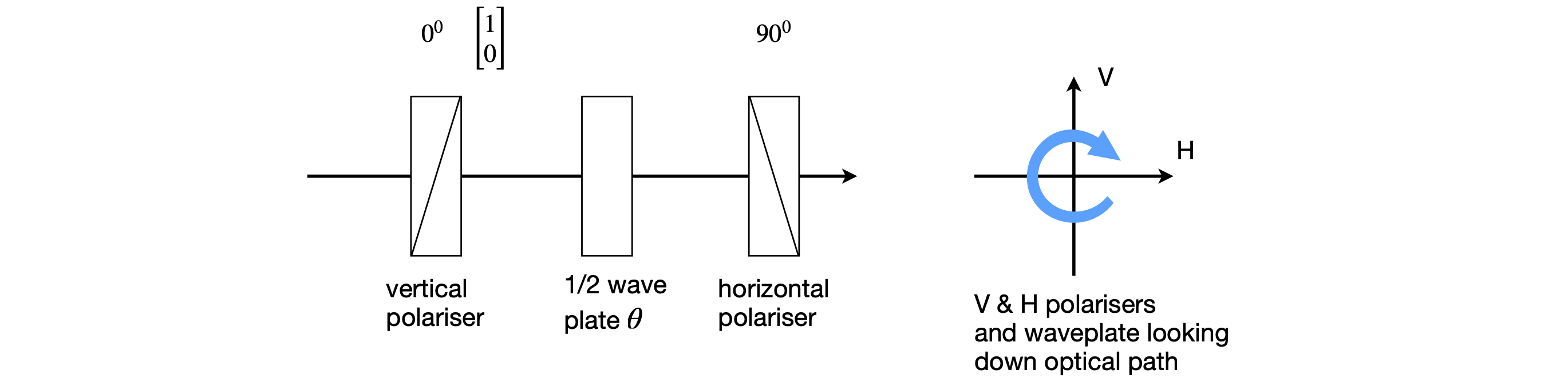# Questions 40 - 44#

## Q40 Linear polarisation#

A beam of linearly polarized light with amplitude components V and H is passed through a polarizing cube set to pass vertical polarized light, $$\theta=0$$. The light is either reflected or transmitted by the cube.

(a) How much light is transmitted and how much reflected when the polarizer is at $$0^\text{o}$$?

(b) How much when at $$45^\text{o}$$?

Strategy: By the nature of the cube, we are told that no photons are absorbed thus the total number remains constant and the number reflected must be the total less those transmitted. Remember that intensity is always amplitude squared.

## Q41 Half-wave plate#

A half-wave plate is set at some arbitrary angle $$\varphi$$. Show that the angle $$\psi$$ that the polarization is rotated to is twice the angle $$\varphi$$ the wave-plate is set at. What is the nature of the resultant beam in each case and what is its intensity?

## Q42 Polarised light#

Vertically polarized light of amplitude V is passed through a quarter-wave plate

(a) with its fast axis at $$\theta = 0$$;

(b) at $$\theta = 45^\text{o}$$, and

(c) at some arbitrary angle $$\theta$$.

What is the nature of the resultant beam in each case?

## Q43 Polariser#

A beam of vertical, linearly polarized laser light of unit amplitude is passed into a linear polarizer whose angle $$\theta$$ is rotated from $$0 \to 180^\text{o}$$ and the transmitted light measured on a photodiode. Draw a diagram of the experiment then calculate:

(a) how the intensity of the light transmitted by the polarizer varies with $$\theta$$

(b) its polarization at any given angle.

Strategy: Use the diagram of the experiment to work out what matrices are used and in what order. Next, calculate the answer using the matrices for linear polarized light and the matrix for a linear polarizer. Make the input polarization in the vertical direction and this has the matrix $$\displaystyle \begin{bmatrix} 1 \\0 \end{bmatrix}$$. The intensity is the dot product of the resulting column vector with itself, and to make the row vector we must make the Hermitian transpose by replacing any $$i =\sqrt{(-1)}$$ with $$-i$$ in the transpose, if a complex quantity is present.

## Q44 half-wave plate#

A half-wave plate is placed between a pair of crossed linear polarizers. A beam of light of unit amplitude is transmitted by the first polarizer set at $$0^\text{o}$$ and is rotated by a half wave plate set at $$\theta$$.

(a) What is the intensity transmitted by the second polarizer for any angle $$\theta$$ of the half wave plate?

(b) Show that the maximum transmitted intensity occurs when the half-wave plate is set at $$\pm 45^\text{o}$$.

(c) What is the intensity variation if the wave-plate is restricted to small angles from the vertical?

(d) Generalize part (a) to any linear polarizer angles $$\alpha$$ and $$\beta$$, and wave-plate $$\theta$$ angles and arbitrary input intensities $$V$$ and $$H$$. Calculate the result of part (a) again.

Use python/Sympy to do the matrix multiplications if you wish.

Strategy: Draw out the arrangement first to know the order of the optical elements and the polarization directions. As the beam is passed through the linear polarizer set at $$0^\text{o}$$, the output from this must be vertically polarized light with matrix $$\displaystyle \begin{bmatrix} 1 \\0 \end{bmatrix}$$ so we can use this to start the calculation. As the polarizers are crossed, the second is at $$90^\text{o}$$ to the first.Figure 52. Scheme for Q44.